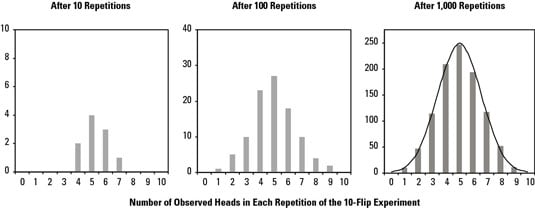##### Six Sigma For DummiesWhat happens when you take repeated samples from the same population? This idea is important when you use the central limit theorem for Six Sigma. Imagine flipping a coin ten times and counting the number of heads you get. The laws of probability say that you have a 50-50 chance of getting heads on any single toss. If you toss the coin ten times, you’d expect to get five heads.

Go ahead and pull a coin out of your pocket and try this experiment if you want. You may not get the expected five heads after flipping the coin ten times. You may get only three heads. Or maybe you get six. After each experiment repetition (sample), the number of heads out of the ten flips was counted. The experiment was repeated 10, then 100, and finally 1,000 times.

This coin flip experiment is analogous to any situation where you take a sample of data from a population — like taking a sample of measurements from a process and calculating the average. Two important facts arise from that you can generalize to any sampling situation:• Repetitions of the measurement event produce different outcome results. That is, the result is variable from sample to sample. In the coin-flipping experiment, not every repetition of the ten-flip series produced the expected five heads. The same is true if you repeatedly take a five-point average of the thickness of paper coming out of a paper mill.

• This resulting measurement, or sampling distribution, is normally distributed. The variation is also centered on the expected outcome. And the more repetitions you make, the closer and closer the sampling variation gets to a perfectly normal distribution.

Statisticians call repeated measurements of a characteristic or a process samples. So the variation that occurs in repeated sampling events they call its sampling distribution.

The sample measurements themselves aren’t the only things that vary when you’re dealing with repeated samples. Statisticians have refined and honed technical definitions of what is called the central limit theorem. Although each definition is equally mysterious, they say the same basic thing: When you calculate statistics on a sample , repeating those calculations on another sample from the same population will always give you a slightly different result.

Additionally, the collection of repeated calculated results will always have a distribution itself. This sampling variation follows a normal bell curve centered on the true variation of the underlying population. Further, the width of the sampling distribution depends on how many measurements you take in each sample. The larger your sample size, the narrower the sampling variation.

Although statisticians often have a difficult time explaining the central limit theorem, its power and utility are nevertheless remarkable. The results of the central limit theorem allow you to predict the bounds of the future and to quantify the risks of the past.